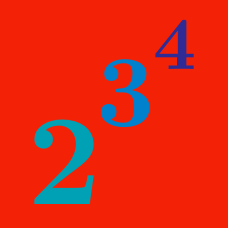Algebra

# Rules of Exponents: Level 1 Challenges

$\large \color{red}{2000}\left(\color{blue}{2000}^{\color{green}{2000}}\right) = \, \color{purple}?$

If $$-1 < x < 0,$$ then what is the correct ordering of $$x, x^2, x^3,$$ and $$x^4?$$

True or false:

$\huge \displaystyle \sqrt{3^{3^3}} = 3^3 .$

Evaluate $\large 16^{0.125} - (0.5)^{-0.5}$

$\huge 2^{2^{2^2}} = \ ?$

×